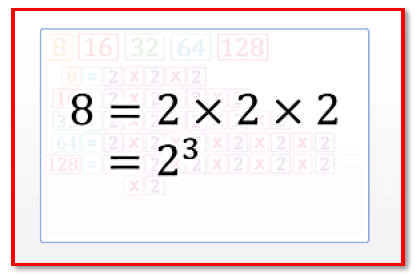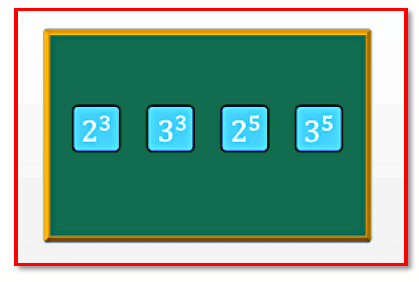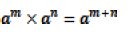Courses

# Chapter Notes - Exponents and Powers Class 7 Notes | EduRev

## Mathematics (Maths) Class 7

Created by: Praveen Kumar

## Class 7 : Chapter Notes - Exponents and Powers Class 7 Notes | EduRev

The document Chapter Notes - Exponents and Powers Class 7 Notes | EduRev is a part of the Class 7 Course Mathematics (Maths) Class 7.
All you need of Class 7 at this link: Class 7

13. Exponents and Powers

Exponents and PowersAn exponent or power is a mathematical representation that indicates the number of times that a number is multiplied by itself.

An exponent or power is a mathematical representation that indicates the number of times that a number is multiplied by itself.

If a number is multiplied by itself m times, then it can be written as: a x a x a x a x a...m times = am

Here, a is called the base, and m is called the exponent, power or index.

Numbers raised to the power of two are called square numbers.

Square numbers are also read as two-square, three-square, four-square, five-square, and so on.

Numbers raised to the power of three are called cube numbers.

Cube numbers are also read as two-cube, three-cube, four-cube, five-cube, and so on.

Negative numbers can also be written using exponents.

If an = b, where a and b are integers and n is a natural number, then an is called the exponential form of b.

The factors of a product can be expressed as the powers of the prime factors of 100.

This form of expressing numbers using exponents is called the prime factor product form of exponents.

Page 52

Even if we interchange the order of the factors, the value remains the same.

So a raised to the power of x multiplied by b raised to the power of y, is the same as b raised to the power of y multiplied by a raised to the power of x.

The value of an exponential number with a negative base raised to the power of an even number is positive.

If the base of two exponential numbers is the same, then the number with the greater exponent is greater than the number with the smaller exponent.

A number can be expressed as a decimal number between 1.0 and 10.0, including 1.0, multiplied by a power of 10. Such a form of a number is known as its standard form.

Laws of ExponentsWhen numbers with the same base are multiplied, the power of the product.

When numbers with the same base are multiplied, the power of the product is equal to the sum of the powers of the numbers.

More precisely if m and n are whole numbers then,When numbers with the same base are divided, then the power of the quotient is equal to the difference between the powers of the dividend and the divisor. That is, if is a non-zero integer, and m and are whole numbers then,  am ÷ an = am-n

The other laws of exponents are as follows:

1) am ÷ an = am-n  , where> are non - zero integers, and m is a whole number.

2) am ÷ an = am-n  , where are non - zero integers, and m is a whole number.

3)  am ÷ an = am-n  ,where> a is a non-zero integer, and m and n are whole numbers.

4)  a0 = 1,  The value ,where> a is a non-zero integer.

5) ,where> a is a non - zero integer, and m and n are whole numbers.

Offer running on EduRev: Apply code STAYHOME200 to get INR 200 off on our premium plan EduRev Infinity!

,

,

,

,

,

,

,

,

,

,

,

,

,

,

,

,

,

,

,

,

,

;